# Algebra 1 : Number Lines and Absolute Value

## Example Questions

← Previous 1 3 4

### Example Question #1 : Number Lines And Absolute Value

Plot the fraction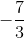on the number line.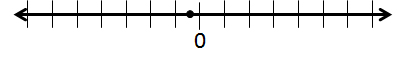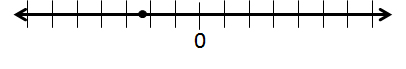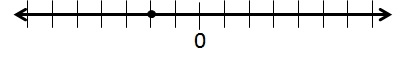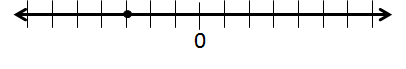Explanation:

The fractionis less thanand greater than, so it must fall between those points on the number line. Negative numbers are to the left ofwhile positive numbers are to the right.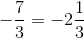Becauseis less than, the point must be closer tothan.

### Example Question #1 : How To Find Absolute Value

What number or numbers have absolute value?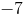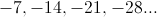No such numbers exist.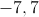No such numbers exist.

Explanation:

The absolute value of every real number is nonnegative, so no number with absolute valuecan exist.

### Example Question #2 : How To Find Absolute Value

What number or numbers have absolute value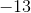?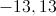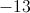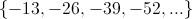No such numbers exist.

No such numbers exist.

Explanation:

The absolute value of every real number is nonnegative, so no number with absolute valuecan exist.

### Example Question #3 : How To Find Absolute Value

What number or numbers have absolute value 29?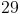No such number exists.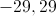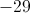Explanation:

The absolute value of any positive number is the number itself, so 29 has 29 as an absolute value. Also, the absolute value of a negative number is its (positive) opposite, so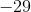also has 29 as an absolute value.

### Example Question #4 : How To Find Absolute Value

What number or numbers have absolute value 11?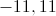No such numbers exist.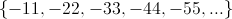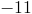Explanation:

The absolute value of any positive number is the number itself, so 11 has 11 as an absolute value. Also, the absolute value of a negative number is its (positive) opposite, so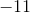also has 11 as an absolute value.

### Example Question #1 : How To Find Absolute Value

Evaluate the expression.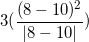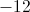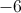Explanation:First, simplify the terms in parentheses and absolute value.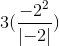Remember that the absolute value of a negative term is positive.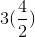Multiply and simplify.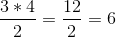### Example Question #6 : How To Find Absolute Value

Solve for.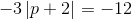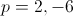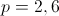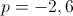A solution does not exist.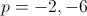Explanation:

Divide both sides by.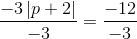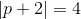Remove the absolute value brackets by setting the expression equal to both the positive and negative values of 4: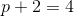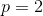or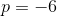### Example Question #1 : How To Find Absolute Value

Mr. Smith and his wife went on a trip, they travelledmiles towards their destination, however on the way there they had to turn around and drivemiles away from their destination to get Mr. Smith's wallet from the store. After getting his wallet they drovemore miles to reach their destination. What is the total number of miles that the family covered?

Not enough information.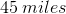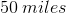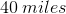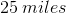Explanation:

Even though the Smiths travelled backwards you still have to add the positive number to the equation. So it looks like this: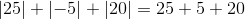which equals### Example Question #8 : How To Find Absolute Value

Simplify the following equation: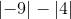Explanation:

It is important to understand that the absolute value is the distance froma number is.

To simply this equation you need to simplify the absolute values first.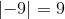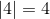Then simplify the function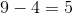### Example Question #9 : How To Find Absolute Value

Solve: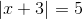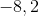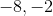Explanation:

In order to solve this absolute value, we will need to split up the absolute value in its positive and negative solution.

Break up the absolute value and rewrite the terms for the positive solution and solve for.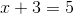For the negative solution, split up the absolute value and add a negative in front of the quantity which was contained by the absolute value.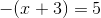Divide by negative one on both sides.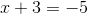Subtract three on both sides.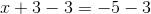Simplify both sides.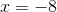The answers to this question are:← Previous 1 3 4